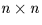Next: SLA_SVDSOL - Solution Vector from SVD
Up: SUBPROGRAM SPECIFICATIONS
Previous: SLA_SVD - Singular Value Decomposition

## SLA_SVDCOV - Covariance Matrix from SVD

ACTION:
From the W and V matrices from the SVD factorization of a matrix (as obtained from the sla_SVD routine), obtain the covariance matrix.

CALL:
CALL sla_SVDCOV (N, NP, NC, W, V, WORK, CVM)

GIVEN:

 I n, the number of rows and columns in matrices W and V N NP I first dimension of array containingmatrix V NC I first dimension of array CVM W D(N)diagonal matrix W (diagonal elements only) V D(NP,NP) array containingorthogonal matrix V

RETURNED:

 D(N) WORK workspace CVM D(NC,NC) array to receive covariance matrix

REFERENCE:
Numerical Recipes, section 14.3.Next: SLA_SVDSOL - Solution Vector from SVD
Up: SUBPROGRAM SPECIFICATIONS
Previous: SLA_SVD - Singular Value Decomposition

SLALIB --- Positional Astronomy Library